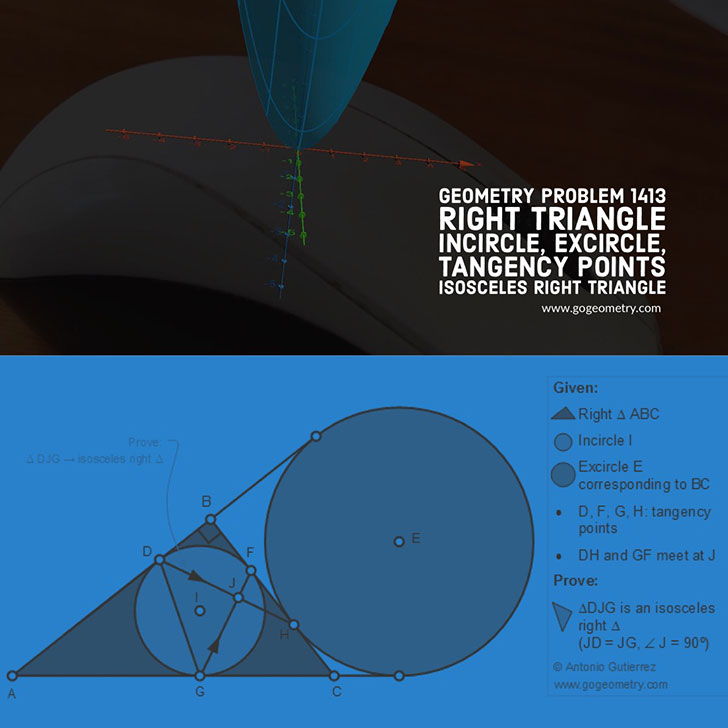# Geometry Problem 1413: Right Triangle, Incircle, Excircle, Tangency Points, Isosceles Right Triangle

### Proposition

The figure below shows a right triangle ABC (angle B = 90 degree) with the incircle I tangent to AB, BC, and AC at D, F, and G, respectively. The excircle E corresponding to BC is tangent to BC at H. DH and GF meet at J. Prove that the triangle DGJ is an isosceles right triangle.### Geometric Art: Poster of problem 1413### Geometric Art: Hyperbolic Kaleidoscope of problem 1413 using iPad Apps